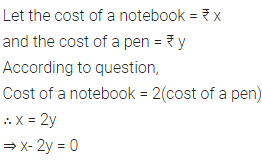# Write a linear equation in two variables to represent this statement

The cost of a notebook is twice the cost of a pen. Write a linear equation in two variables to represent this statement.
(Take the cost of a notebook to be ₹ x and that of a pen to be ₹ y)